1. /
2. CBSE
3. /
4. Class 12
5. /
6. Mathematics
7. /
8. CBSE Question Paper 2015...

# CBSE Question Paper 2015 class 12 Mathematics

CBSE Question Paper 2015 class 12 Mathematics conducted by Central Board of Secondary Education, New Delhi in the month of March 2015. CBSE previous year question papers with solution are available in myCBSEguide mobile app and cbse guide website. The Best CBSE App for students and teachers is myCBSEguide which provides complete study material and practice papers to cbse schools in India and abroad.

CBSE Question Paper 2015 class 12 Mathematics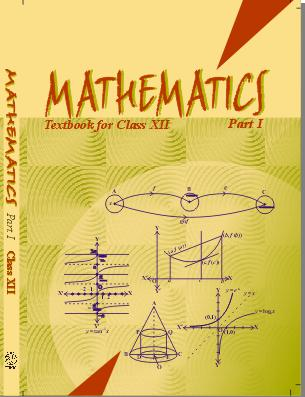## Class 12 Mathematics list of chapters

1. Relations and Functions
2. Inverse Trigonometric Functions
3. Matrices
4. Determinants
5. Continuity and Differentiability
6. Application of Derivatives
7. Integrals
8. Application of Integrals
9. Differential Equations
10. Vector Algebra
11. Three Dimensional Geometry
12. Linear Programming
13. Probability

## CBSE Question Paper 2015 class 12 Mathematics

### General Instructions:

(i) There ae total of 26 questions in all. All questions are compulsory.

(ii) The question paper consists of 26 questions divided into three sections A, B and C. Section ‘A’ comprises of 6 questions of one mark each. Section ‘B’ comprises of 13 questions of four marks each. Section ‘C’ comprises of 7 question of six marks each.

(iii) All questions in Section ‘A’ are to be answered in one word, one sentence or as per the exact requirement of the question.

(iv) There is no overall choice. However, an internal choice has been provided in 4 questions of four marks each and 2 questions of six marks each. You have to attempt only one of the alternatives in all such questions.

### SECTION: A

1. For what value of x, the matrix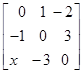is skew symmetric matrix?

2. If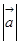=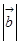=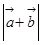=1, then find the value of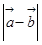.

3. Write the equation of a line perpendicular to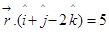and passing through point (1, 0 , 3).

4. If= 3 and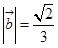, for what angle between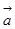and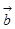,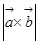=1 ?

5. Write the integrating factor of the differential equation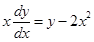.

6. Write the sum of the order and degree of the differential equation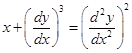.

### SECTION: B

7. Prove that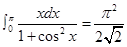OR

Prove that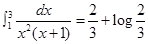8. Evaluate: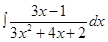9. Evaluate: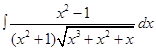10. Let,,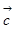be unit vectors such that.=.= 0 and the angle betweenandis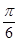, prove that: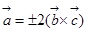11. If y =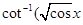=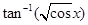, then prove that: sin y =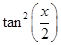### OR

Solve the equation: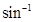(1-x)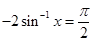12. If A =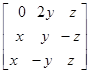and A’A = I, then find the value of x, y and z.

13. Using properties of determinants, prove that: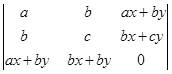=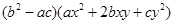14. If x=a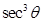, y = a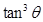, then find the value of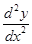at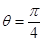.

OR

Find the value of ‘k’ for which the function defined as:

F(x) =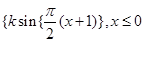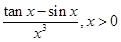Is continuous at x=0.

15. A trust fund has Rs. 60000 that must be invested in two different types of bonds. The first bond pays 3% interest per year and the second bond pays 5% interest per year. Using matrix multiplication, determine how to divide Rs. 60000 among the two types of bonds, if the trust fund must obtain an annual total interest of Rs. 2000.

These are questions only. To view and download complete question paper with solution install myCBSEguide App from google play store or login to our student dashboard.

## Class 12 Mathematics Last Year Paper

Download Mathematics Last Year Paper 2015 with solution from best CBSE App the myCBSEguide. CBSE class 12 Mathematics question paper 2015 in PDF format with solution will help you to understand the latest question paper pattern and marking scheme of the CBSE board examination. You will get to know the difficulty level of the question paper. CBSE question papers 2015 for class 12 Mathematics have 29 questions with solution.

## Previous Year Questions Papers 2015

CBSE question papers 2018, 2017, 2016, 2015, 2014, 2013, 2012, 2011, 2010, 209, 2008, 2007, 2006, 2005 and so on for all the subjects are available under this download link. Practicing real question paper certainly helps students to get confidence and improve performance in weak areas.

To download CBSE Question Paper 2015 class 12 Accountancy, Chemistry, Physics, History, Political Science, Economics, Geography, Computer Science, Home Science, Accountancy, Business Studies and Home Science; do check myCBSEguide app or website. myCBSEguide provides sample papers with solution, test papers for chapter-wise practice, NCERT solutions, NCERT Exemplar solutions, quick revision notes for ready reference, CBSE guess papers and CBSE important question papers. Sample Paper all are made available through the best app for CBSE students and myCBSEguide website.### Test Generator

Create question paper PDF and online tests with your own name & logo in minutes.### myCBSEguide

Question Bank, Mock Tests, Exam Papers, NCERT Solutions, Sample Papers, Notes